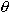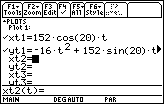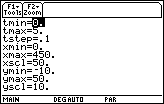Module 12 - Particle Motion and Parametric Models Introduction | Lesson 1 | Lesson 2 | Lesson 3 | Self-Test Lesson 12.3: Motion in a Plane Motion along a line was modeled in Lesson 12.1 where the particle's movement was given as polynomial. Harmonic motion was modeled in Lesson 12.2. In this lesson motion in a plane will be investigated. Stating the Problem A baseball is hit at an angle of 20° above the horizon with an initial velocity of 152 ft/sec and an initial height of 3 feet off the ground. Will the ball clear a 10 foot wall at a distance of 415 feet away from home plate? Modeling the Motion Letting v represent the balls's initial velocity, h represent the initial height,represent the angle at which the ball is hit, and t represent time in seconds since the ball was struck, movement of the ball in the plane can be modeled using the parametric equations below. x(t) = v t cosy(t) = –16t2 + vtsin+ h In the given problem v = 152, h = 3, and= 20. Set xt1 = 152 cos(20) t Set yt1 = -16t^2 + 152 sin(20) t + 3Select DEGREE for the Angle setting in the Mode dialog box Set the Viewing Window values shown below12.3.1 Graph the equations and decide if the ball clears the fence. Click here for the answer. Finding Maximum Height The height of the ball is an inverted parabola, so the maximum occurs at the vertex or local maximum of the height. To find the maximum height of the ball, find the time when the derivative of the height is equal to zero and evaluate the height function with that value. Enter solve(d(yt1(t),t)=0,t) | 0 ©Copyright 2007 All rights reserved. | Trademarks | Privacy Policy | Link Policy